# Precalculus : Solve Angular Velocity Problems

## Example Questions

### Example Question #1 : Solve Angular Velocity Problems

If a ball is travelling in a circle of diameter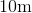with velocity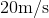, find the angular velocity of the ball.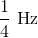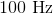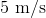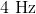Explanation:

Using the equation,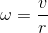where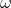=angular velocity,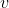=linear velocity, and=radius of the circle.

In this case the radius is 5 (half of the diameter) and linear velocity is 20 m/s.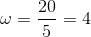.

### Example Question #1 : Solve Angular Velocity Problems

Suppose a car tire rotatestimes a second. The tire has a diameter ofinches. Find the angular velocity in radians per second.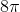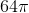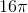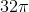Explanation:

Write the formula for angular velocity.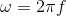The frequency of the tire is 8 revolutions per second. The radius is not used.

Substitute the frequency and solve.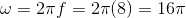### Example Question #1 : Solve Angular Velocity Problems

What is the angular velocity of a spinning top if it travelsradians in a third of a second?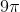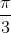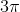Explanation:

Write the formula for average velocity.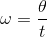The units of omega is radians per second.

Substitute the givens and solve for omega.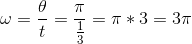### Example Question #1 : Solve Angular Velocity Problems

A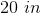diamter tire on a car makes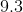revolutions per second. Find the angular speed of the car.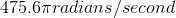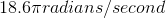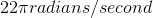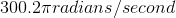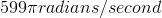Explanation:

Recall that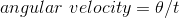.

Since the tire revolves 9.3 times/second it would seem that the tire would rotate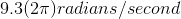or.

We useto indicate that the tire is rolling 360 degrees orradians each revolution (as it should).

Thus,is your final answer.

Note that radians is JUST a different way of writing degrees. The higher numbers in the answers above are all measures around the actual linear speed of the tire, not the angular speed.

### Example Question #1 : Angular And Linear Velocity

A car wheel of radius 20 inches rotates at 8 revolutions per second on the highway. What is the angular speed of the tire?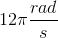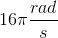None of these.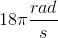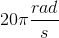Explanation:

Angular speed is the same as linear speed, but instead of distance per unit time we use degrees or radians. Any object traveling has both linear and angular speed (though objects only have angular speed when they are rotating).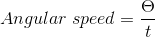Since our tire completes 8 revolutions per second we multiply bysince a full rotation (360°) equals.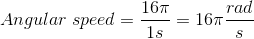### All Precalculus Resources